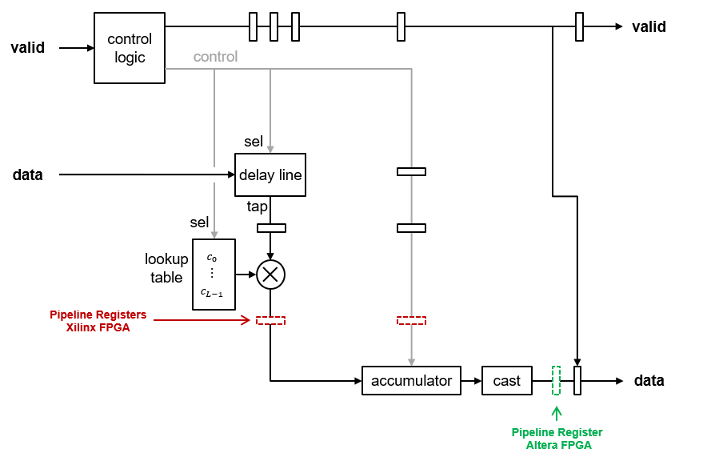## FIR Filter Architectures for FPGAs and ASICs

The Discrete FIR Filter, FIR Decimator, FIR Interpolator, Farrow Rate Converter, Channelizer, and Channel Synthesizer blocks all use the same FIR filter architectures to implement their algorithms.

These blocks provide filter implementations that make trade-offs between resources and throughput. The filter implementations also consider vendor-specific hardware details of the DSP blocks when adding pipeline registers to the architecture. These differences in pipeline register locations help fit the filter design to the DSP blocks on the FPGA. For a filter implementation that matches multipliers, pipeline registers, and pre-adders to the DSP configuration of your FPGA vendor, specify your target device when you generate HDL code.

The filter implementations remove multipliers for zero-valued coefficients, such as in half-band filters and Hilbert transforms. When you use scalar input data, the filters share multipliers for symmetric and antisymmetric coefficients. Frame-based filters do not support symmetry optimization.

The FIR filter implementations implement efficient complex multiplier architectures and support frame based input by using polyphase filters that share hardware resources across subfilters .

The architecture diagrams on this page assume a transfer function that has L coefficients (before optimizations that share multipliers for symmetric or antisymmetric or remove multipliers for zero-valued coefficients). N represents the number of cycles between valid input samples.

Filter StructureBlocksSettings
Fully Parallel Systolic Architecture
• Discrete FIR Filter

• Farrow Rate Converter

• Channelizer

• Channel Synthesizer

• FIR Decimator

• FIR Interpolator

• For Discrete FIR Filter, Farrow Rate Converter, Channelizer, and Channel Synthesizer — Set Filter structure to `Direct form systolic`.

• For FIR Decimator and FIR Interpolator — Set Filter structure to `Direct form systolic` and Number of Cycles to 1.

Fully Parallel Transposed Architecture
• Discrete FIR Filter

• Farrow Rate Converter

• Channelizer

• Channel Synthesizer

• FIR Decimator

• FIR Interpolator

Set Filter structure to `Direct form transposed`.
Partly Serial Systolic Architecture (1 < N < L)
• Discrete FIR Filter

• Farrow Rate Converter

• FIR Decimator

• FIR Interpolator

• For Discrete FIR Filter — Set Filter structure to `Partly serial systolic` and specify a serialization factor by either cycles or multipliers that results in 1<N < L.

• For Farrow Rate Converter, FIR Decimator, and FIR Interpolator — Set Filter structure to `Direct form systolic` and Minimum number of cycles between valid input samples to a value greater than 1 but less than L.

Fully Serial Systolic Architecture (N ≥ L)
• Discrete FIR Filter

• Farrow Rate Converter

• FIR Decimator

• FIR Interpolator

• For Discrete FIR Filter — Set Filter structure to `Partly serial systolic` and specify a serialization factor by cycles such that NL, or set the Number of multipliers to `1`.

• For Farrow Rate Converter, FIR Decimator, and FIR Interpolator — Set Filter structure to `Direct form systolic` and Minimum number of cycles between valid input samples to a value greater than L.

### Complex Multipliers

If either data or coefficients are complex but not both, the filter blocks implement one filter to calculate the real output and a second filter to calculate the imaginary part. This implementation results in two multipliers for each filter tap.

When both the data and coefficients are complex, the block implements three filters in parallel. The diagram shows the filter implementation for complex input data X = Xr+i×Xi and complex coefficients W = Wr+i×Wi.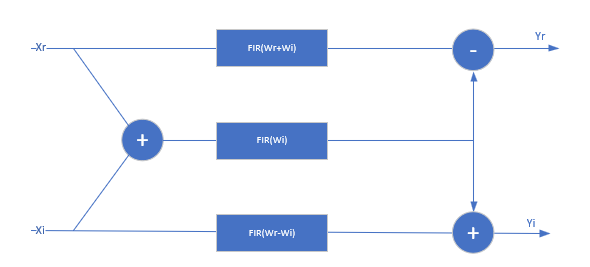When you specify coefficients from a parameter, Wr + Wi and Wr-Wi are pre-calculated, so this implementation uses 3 DSP blocks for each filter tap, plus the input adder and two output adders. The input to each filter tap multiplier grows by one bit.

When you use programmable coefficients, the filter uses 2 more adders for each filter tap. These adders calculate the coefficients Wr + Wi and Wr-Wi.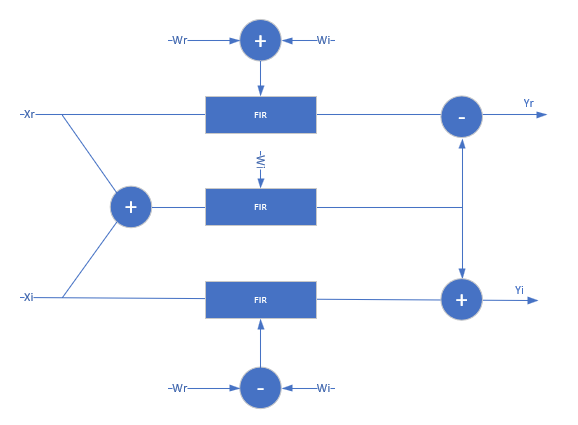### Frame-Based Input Data

The Discrete FIR Filter, FIR Decimator, FIR Interpolator, Channelizer, and Channel Synthesizer blocks accept frame-based input data to support gigasamples-per-second throughput. When you apply frame-based input data, the FIR filter implements a polyphase decomposition of your filter coefficients into V subfilters, where V is the size of the input vector. The frame-based filter increases throughput and uses more hardware resources than the scalar-input case. Frame-based filters do not implement symmetry optimization.

For a filter with a 1-by-2 input vector, `[Y0 Y1]`, the diagram shows the polyphase decomposition into two subfilters that implement this equation.

`$\begin{array}{l}{Y}_{0}\left(z\right)={X}_{0}\left(z\right){H}_{0}\left(z\right)+{z}^{-1}{X}_{1}\left(z\right){H}_{1}\left(z\right)\\ {Y}_{1}\left(z\right)={X}_{0}\left(z\right){H}_{1}\left(z\right)+{X}_{1}\left(z\right){H}_{0}\left(z\right)\end{array}$`Each subfilter takes scalar input and is implemented with the architecture you selected, either `Direct form systolic` or `Direct form transposed`. If the subfilters have different latencies due to different numbers of coefficients, or zero-value coefficient optimization, then the implementation includes internal delays to align the output samples. You cannot use frame-based input with the serial systolic architecture.

When you use frame-based input with programmable coefficients, the output may not match sample-for-sample with the output in scalar mode. This difference is because of the internal timing of applying each sample in the input vector to the subfilters. Changes in the input coefficients effectively occur at different individual input samples than they do in scalar mode.

### Fully Parallel Systolic Architecture

This filter architecture is a fully parallel systolic architecture with optimizations for symmetry or anti-symmetry and zero-valued coefficients. The latency depends on the coefficient symmetry and is displayed on the block icon.

When symmetric pairs of coefficients have equal absolute values, they share one DSP block. This pair-sharing enables the implementation to use the pre-adder in Xilinx® and Intel® DSP blocks. The top half of the diagram shows a symmetric filter without the pair coefficient optimization. The bottom half of the diagram shows the architecture using the pair coefficient optimization.### Fully Parallel Transposed Architecture

The fully parallel transposed architecture minimizes multipliers by sharing multipliers for any two or more coefficients that have equal absolute values. It also removes multipliers for zero-valued coefficients. The latency of the filter is six cycles when you use scalar input. This latency does not change with coefficient values.

The top half of the diagram shows the theoretical architecture for a partly-symmetric filter without the equal-absolute-value coefficient optimization. The bottom half of the diagram shows the transposed architecture as implemented using the equal-value coefficient optimization. If the coefficients are antisymmetric, the output adder becomes a subtraction.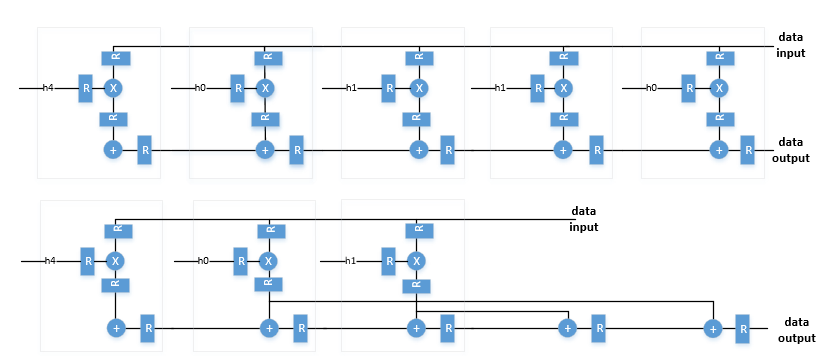### Partly Serial Systolic Architecture (1 < `N` < L)

The partly serial implementation uses `M = ceil(L/N)` systolic cells. Each cell consists of a delay line, coefficient lookup table, and DSP (multiply-add) block. The coefficients are spread across the M lookup tables. The computation performed by each DSP block is serialized. Input samples to the block must be scalar and at least N cycles apart. The latency of the block is `M + ceil(L/M) + 5`.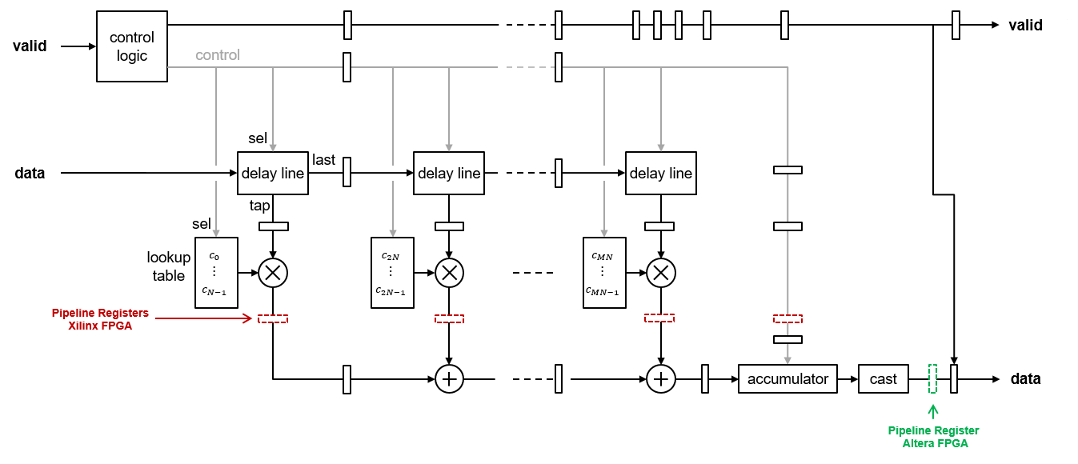If all the coefficients in the lookup table for a multiplier are zeros or powers of two, the implementation does not include that multiplier. The powers of two multiplications are implemented as shifts.

The block implements a RAM-based delay line that uses fewer resources than a register-based implementation. Uninitialized RAM locations can result in `X` values at the start of your HDL simulation. You can avoid `X` values by initializing the RAM from your test bench, or by enabling the Initialize all RAM blocks Configuration Parameter. This parameter sets the RAM locations to`0` for simulation and is ignored by synthesis tools.

### Fully Serial Systolic Architecture (N ≥ L)

When you choose a serialization factor such that NL, the block implements a fully serial systolic architecture. For real coefficients and real input, the filter uses a single DSP (multiply-add) block with a delay line and a lookup table for all L coefficients. Input samples must be at least N cycles apart. The latency of the filter is `L + 5`.